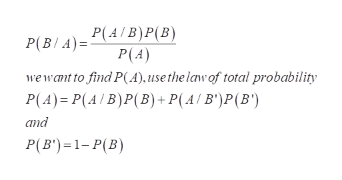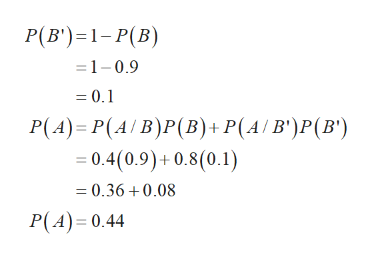# Use Bayes' theorem or a tree diagram to calculate the indicated probability. Round your answer to four decimal places. P(A | B) = .4, P(B) = .9, P(A | B') = .8. Find P(B | A).P(B | A) =

Question
1 views

Use Bayes' theorem or a tree diagram to calculate the indicated probability. Round your answer to four decimal places.

P(A | B) = .4, P(B) = .9, P(A | B') = .8. Find P(B | A).

P(B | A) =

check_circle

Step 1

Given that

P (A/B) = 0.4

P (B) = 0.9

P (A/B’) = 0.8

P (B/A) =?

Step 2

According to Bayes’ theorem, they defined thathelp_outlineImage TranscriptioncloseP(A/B)P(B) P(A) P(B/A) wewant to find P(A), use the law of total probability P(A) P(A B)P(B) + P(A/ B')P(B ') and P(B')1-P(B) fullscreen
Step 3

Substitute the...help_outlineImage TranscriptioncloseP(B') 1-P(B) 1-0.9 = 0.1 P(A) P(AIB)P(B) + P(A / B')P (B') =0.4(0.9)+0.8(0.1) =0.36 0.08 P(A) 0.44 fullscreen

### Want to see the full answer?

See Solution

#### Want to see this answer and more?

Solutions are written by subject experts who are available 24/7. Questions are typically answered within 1 hour.*

See Solution
*Response times may vary by subject and question.
Tagged in

### Basic Probability